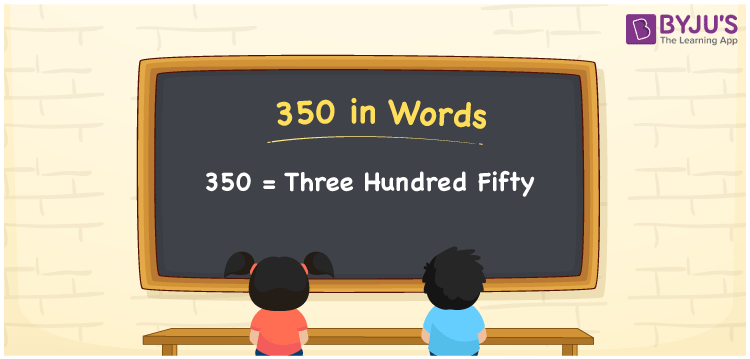# 350 in words

350 in words is written as Three Hundred and Fifty. 350 represents the count or value. The article on Counting Numbers can give you an idea about count or counting. The number 350 is used in expressions that relate to money, days, distance, length, weight and so on. Let us consider an example for 350. “The distance between Bangalore and Mangalore is Three hundred and fifty kilometers.” Another example would be “Apples weighing 350 grams, cost Three Hundred and Fifty Rupees”.

 350 in words Three Hundred and Fifty Three Hundred and Fifty in Numbers 350

## 350 in English Words## How to Write 350 in Words?

We can convert 350 to words using a place value chart. The number 350 has 3 digits, so let’s make a chart that shows the place value up to 3 digits.

 Hundreds Tens Ones 3 5 0

Thus, we can write the expanded form as:

3 × Hundred + 5 × Ten + 0 × One

= 3 × 100 + 5 × 10 + 0 × 1

= 350

= Three Hundred and Fifty.

350 is the natural number that is succeeded by 349 and preceded by 351.

350 in words – Three Hundred and Fifty.

Is 350 an odd number? – No.

Is 350 an even number? – Yes.

Is 350 a perfect square number? – No.

Is 350 a perfect cube number? – No.

Is 350 a prime number? – No.

Is 350 a composite number? – Yes.

## Solved Example

1. Write the number 350 in expanded form

Solution: 3 × 100 + 5 × 10 + 0 × 1

We can write 350 = 300 + 50 + 0

= 3 × 100 + 5 × 10 + 0 × 1

## Frequently Asked Questions on 350 in words

Q1

### How to write the number 350 in words?

350 in words is written as Three Hundred and Fifty.
Q2

### State True or False. 350 is divisible by 3?

False. 350 is not divisible by 3.
Q3

### Is 350 a perfect square number?

No. 350 is not a perfect square number.